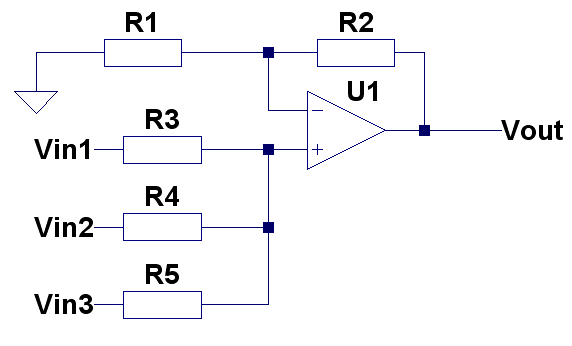# Non-inverting summing amplifier

##Gain = 1+R2/R1

If R3 = R4 = R5,

Vout =Gain*( (Vin1+Vin2+Vin3)/3)

R1||R2 = R3||R4||R5 for minimum offset error due input bias current

For different ratio of input signals … well it gets really complex. Checkout the first link in the link section if you really want to find out.

## Example setup

for Vout = Vin1+Vin2+Vin3
R1=24k
R2 = 12k
R3 = R4 = R5 = 24k

R3||R4||R5 =  R1||R2 = 8k